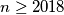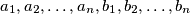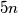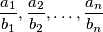### IMO Shortlist 2018 problem N7

Kvaliteta:
Avg: 0,0
Težina:
Avg: 9,0

Let$n \ge 2018$ be an integer, and let$a_1, a_2, \dots, a_n, b_1, b_2, \dots, b_n$ be pairwise distinct positive integers not exceeding$5n$. Suppose that the sequenceforms an arithmetic progression. Prove that the terms of the sequence are equal.

Izvor: https://www.imo-official.org/problems/IMO2018SL.pdf